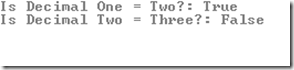# Compare Two Decimal Numbers to N Decimal Places

 Tweet

How do you compare two decimal values to ‘N’ decimal places. So for example, you want to compare two decimal numbers to 5 decimal places. Here’s an example:

C#

`static void Main(string[] args){decimal one   = 23.5456533464M;decimal two   = 23.5456543444M;decimal three = 23.5456223334M;decimal a = Math.Truncate(one * 100000);decimal b = Math.Truncate(two * 100000);decimal c = Math.Truncate(three * 100000);Console.WriteLine("Is Decimal One = Two?: {0}", Equals(a, b));Console.WriteLine("Is Decimal Two = Three?: {0}", Equals(b, c));Console.ReadLine();}`

VB.NET

`Shared Sub Main(ByVal args() As String)Dim one As Decimal = 23.5456533464DDim two As Decimal = 23.5456543444DDim three As Decimal = 23.5456223334DDim a As Decimal = Math.Truncate(one * 100000)Dim b As Decimal = Math.Truncate(two * 100000)Dim c As Decimal = Math.Truncate(three * 100000)Console.WriteLine("Is Decimal One = Two?: {0}", Equals(a, b))Console.WriteLine("Is Decimal Two = Three?: {0}", Equals(b, c))Console.ReadLine()End Sub`

If you observe, we are multiplying each decimal by 5 zeros and considering only the integral part of the decimal using Math.Truncate.

The first two decimal numbers are equal considering the first 5 decimal places .54565. However the third decimal number is .54562, which is not equal to .54565 and hence the value printed is false

OUTPUTWill you give this article a +1 ? Thanks in advance

#### About The AuthorSuprotim Agarwal, Developer Technologies MVP (Microsoft Most Valuable Professional) is the founder and contributor for DevCurry, DotNetCurry and SQLServerCurry. He is the Chief Editor of a Developer Magazine called DNC Magazine. He has also authored two Books - 51 Recipes using jQuery with ASP.NET Controls. and The Absolutely Awesome jQuery CookBook.

Follow him on twitter @suprotimagarwal.